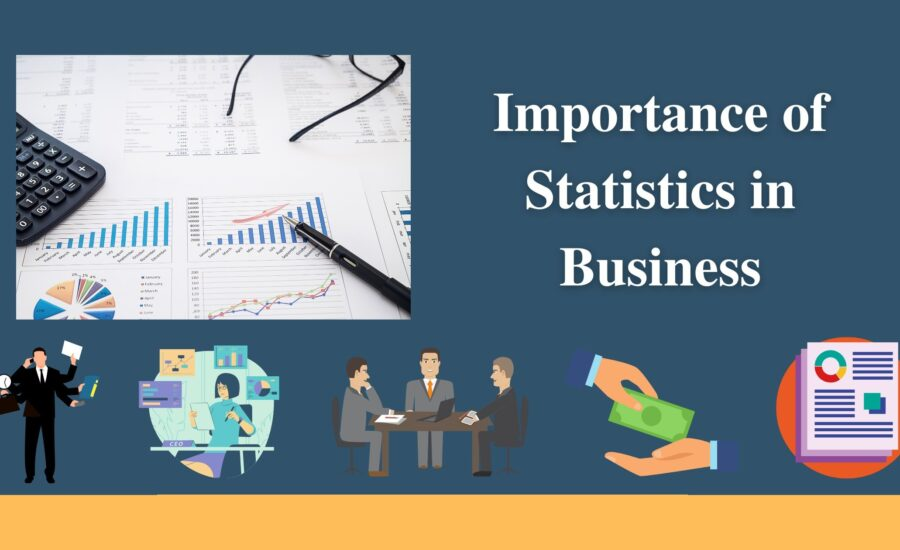S O P H I A C O L L E G E

## Role of Statistics in B.Com - Sophia college

Here!
• Home
• Blogs Role of Statistics in B.Com# What is the role of statistics in B.Com?

• Data Analysis: Statistics enables B.Com students to analyze and interpret data related to business and financial activities. It helps in identifying patterns and trends that can aid in decision-making.
• Market Research: Statistics is an essential tool for conducting market research. B.Com students use statistical methods to analyze consumer behavior, market trends, and preferences.
• Financial Analysis: Statistics helps in analyzing financial data such as revenue, profits, and expenses. B.Com students can use statistical techniques to evaluate the performance of companies and make informed financial decisions.
• Forecasting: Statistics helps in forecasting future trends and patterns for businesses. B.Com students can use statistical models to predict future sales, revenue, and demand.
• Risk Management: Statistics plays a vital role in risk management for businesses. B.Com students can use statistical techniques to identify potential risks and develop strategies to mitigate them.
• Hypothesis Testing: Statistics is used for hypothesis testing in B.Com studies. B.Com students can use statistical inference to test hypotheses and draw conclusions based on data.

## How do statistics help in financial analysis and decision-making for businesses?

• Data Analysis: Statistics enables businesses to analyze financial data such as revenue, expenses, and profits. B.Com students can use statistical methods to identify patterns and trends in financial data, which can aid in decision-making.
• Forecasting: Statistics helps in forecasting future trends and patterns for businesses. B.Com students can use statistical models to predict future sales, revenue, and demand, which can aid in financial planning and decision-making.
• Risk Management: Statistics is an essential tool for managing financial risks. B.Com students can use statistical techniques to identify potential risks and develop strategies to mitigate them. For example, statistical analysis can help businesses determine the likelihood of default on a loan, which can help in making decisions about lending.
• Investment Analysis: Statistics is used in investment analysis to evaluate the performance of investments. B.Com students can use statistical methods to analyze stock market data and make informed investment decisions.
• Financial Reporting: Statistics is used in financial reporting to summarize and present financial data. B.Com students can use statistical techniques to analyze financial statements and prepare reports that provide valuable insights into a company’s financial performance.

## What statistical methods are commonly used in market research for B.Com students?

• Sampling: Sampling is the process of selecting a representative subset of the population to be surveyed. B.Com students use statistical methods to determine the sample size and the sampling method to ensure that the sample is representative of the population.
• Descriptive Statistics: Descriptive statistics are used to summarize and describe the characteristics of the data. B.Com students use measures such as mean, median, mode, standard deviation, and variance to analyze market research data.
• Inferential Statistics: Inferential statistics are used to make predictions about a population based on a sample. B.Com students use statistical techniques such as hypothesis testing, confidence intervals, and regression analysis to draw conclusions from market research data.
• Correlation Analysis: Correlation analysis is used to measure the relationship between two variables. B.Com students use statistical methods to analyze the correlation between different factors that influence consumer behavior, such as price, quality, and brand loyalty.
• Factor Analysis: Factor analysis is used to identify the underlying factors that influence consumer behavior. B.Com students use statistical techniques to identify the underlying factors that influence consumer preferences and behavior.
• Cluster Analysis: Cluster analysis is used to group similar observations or variables together. B.Com students use statistical methods to segment the market based on consumer preferences and behavior.

## How can statistical data be used to evaluate the performance of a company?

• Financial Analysis: Statistical methods can be used to analyze financial data such as revenue, expenses, and profits. By analyzing financial data over time, B.Com students can use statistical methods to identify trends and patterns in a company’s financial performance.
• Ratio Analysis: Ratio analysis is a common technique used to evaluate a company’s financial performance. B.Com students can use statistical ratios such as return on investment (ROI), return on assets (ROA), and debt-to-equity ratio to compare the company’s financial performance with its competitors.
• Benchmarking: Statistical data can be used for benchmarking, which involves comparing a company’s performance with industry standards or best practices. B.Com students can use statistical methods to identify the key performance indicators (KPIs) for their industry and compare a company’s performance with those KPIs.
• Market Research: Statistical data can be used for market research to evaluate a company’s performance in the market. B.Com students can use statistical methods to analyze consumer behavior, preferences, and market trends to evaluate a company’s market share and competitiveness.
• Customer Satisfaction: Statistical data can be used to evaluate a company’s performance in terms of customer satisfaction. B.Com students can use statistical methods to analyze customer feedback and ratings to identify areas of improvement and evaluate the company’s performance.